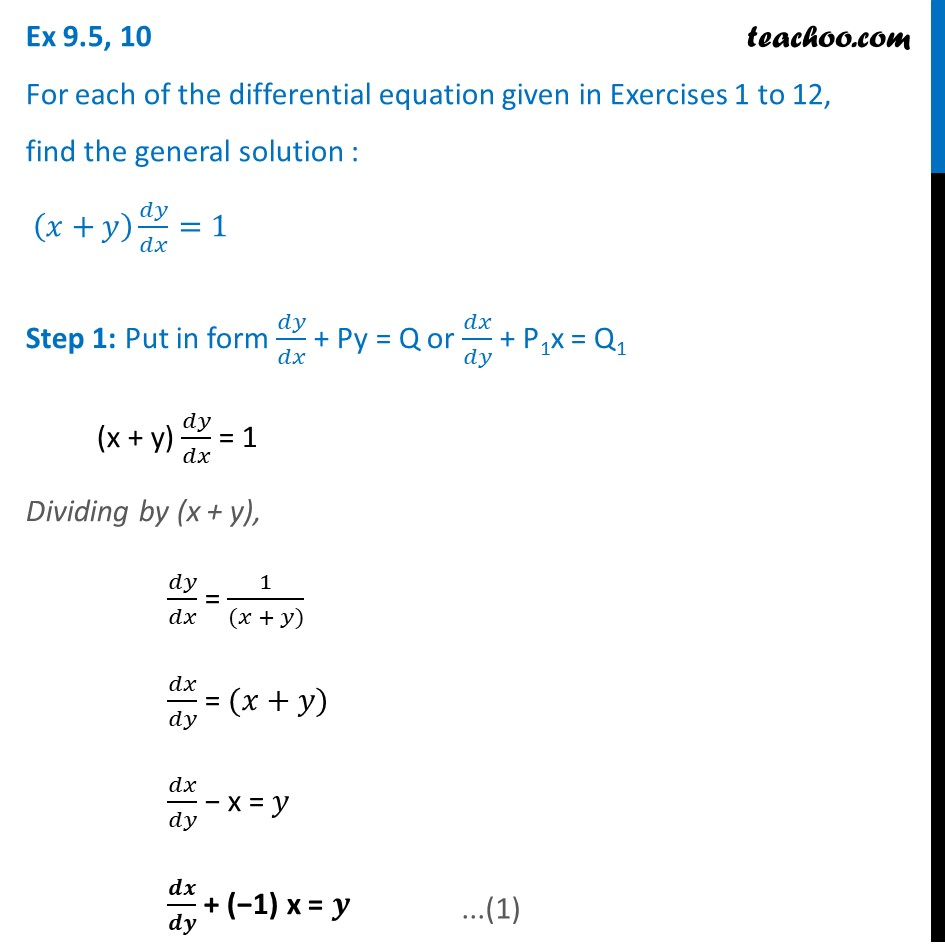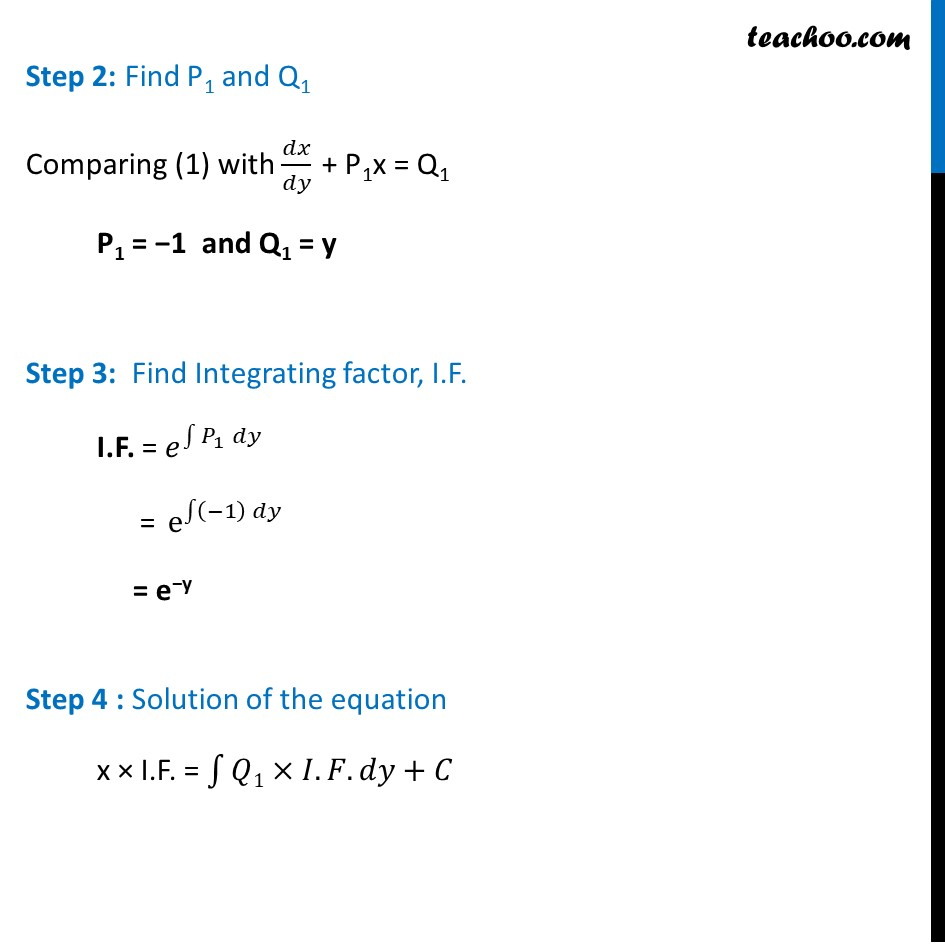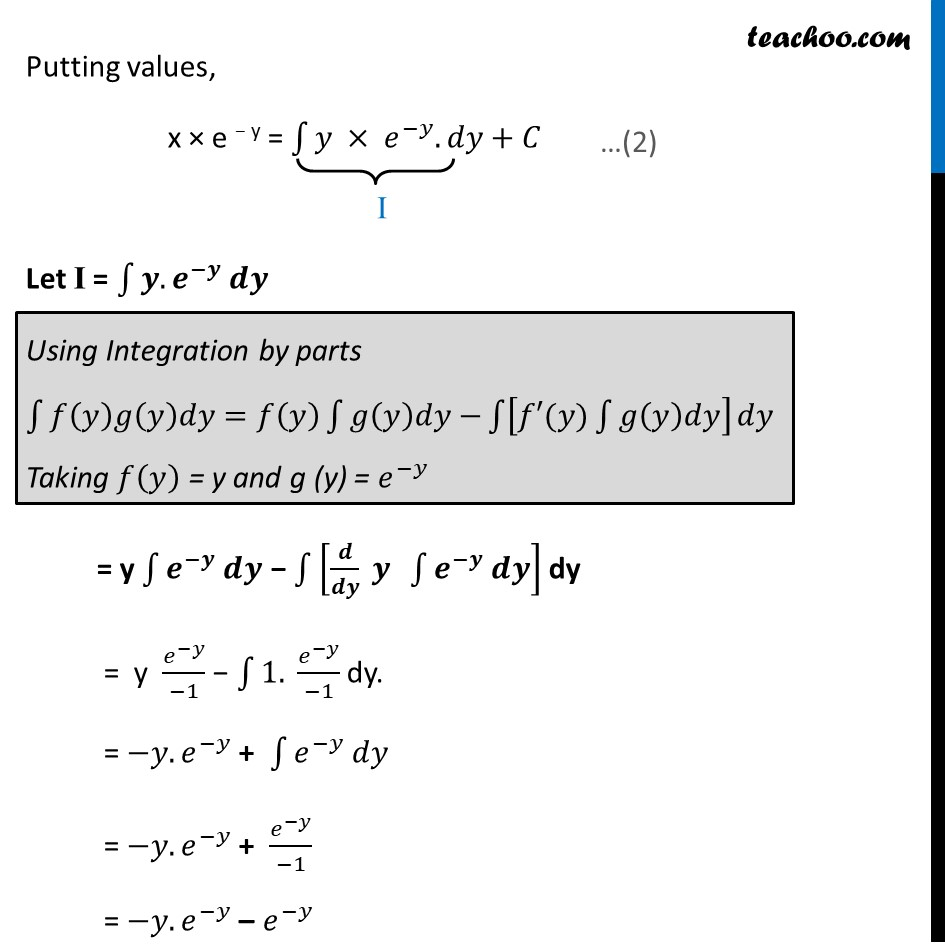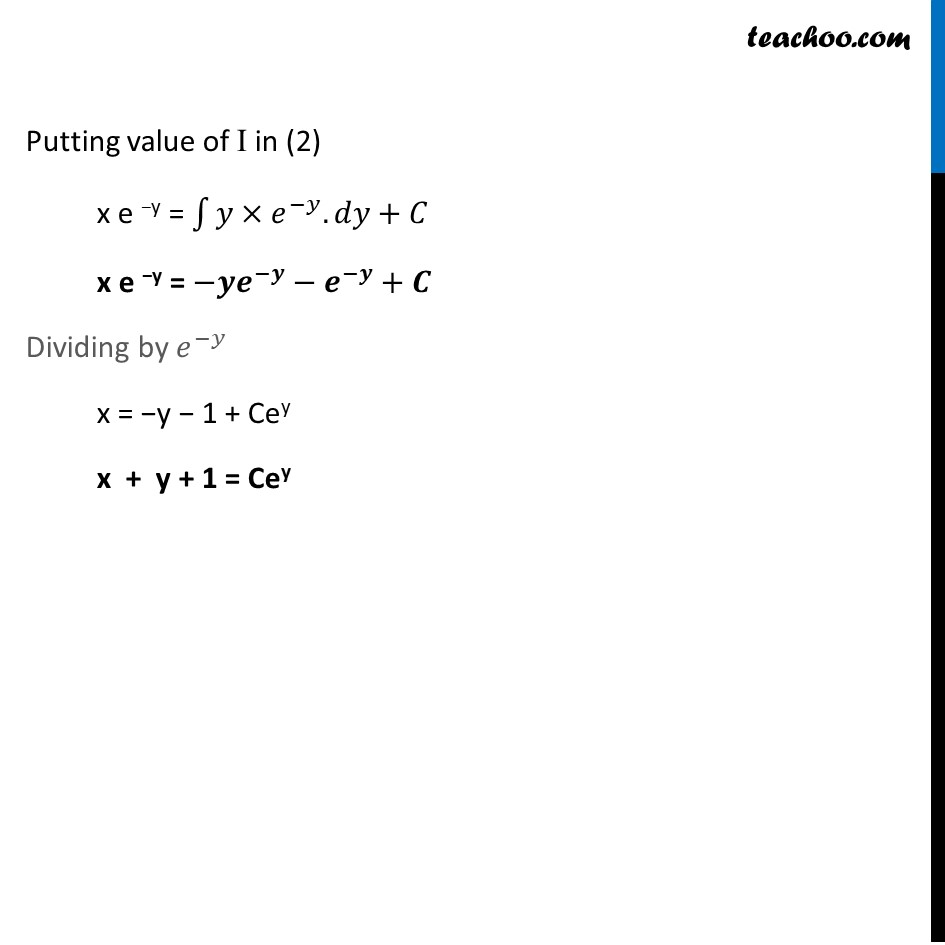Ex 9.5

Chapter 9 Class 12 Differential Equations
Serial order wiseLearn in your speed, with individual attention - Teachoo Maths 1-on-1 Class

### Transcript

Ex 9.5, 10 For each of the differential equation given in Exercises 1 to 12, find the general solution : (𝑥+𝑦) 𝑑𝑦/𝑑𝑥=1 Step 1: Put in form 𝑑𝑦/𝑑𝑥 + Py = Q or 𝑑𝑥/𝑑𝑦 + P1x = Q1 (x + y) 𝑑𝑦/𝑑𝑥 = 1 Dividing by (x + y), 𝑑𝑦/𝑑𝑥 = 1/((𝑥 + 𝑦)) 𝑑𝑥/𝑑𝑦 = (𝑥+𝑦) 𝑑𝑥/𝑑𝑦 − x = 𝑦 𝒅𝒙/𝒅𝒚 + (−1) x = 𝒚 Step 2: Find P1 and Q1 Comparing (1) with 𝑑𝑥/𝑑𝑦 + P1x = Q1 P1 = −1 and Q1 = y Step 3: Find Integrating factor, I.F. I.F. = 𝑒^∫1▒〖𝑃_1 𝑑𝑦〗 = e^∫1▒〖(−1) 𝑑𝑦〗 = e−y Step 4 : Solution of the equation x × I.F. = ∫1▒〖𝑄1×𝐼.𝐹. 𝑑𝑦+𝐶〗 Putting values, x × e − y = ∫1▒〖𝑦 × 𝑒^(−𝑦).〗 𝑑𝑦+𝐶 Let I = ∫1▒〖𝒚.𝒆^(−𝒚) 𝒅𝒚〗 Using Integration by parts ∫1▒〖𝑓(𝑦)𝑔(𝑦)𝑑𝑦=𝑓(𝑦) ∫1▒〖𝑔(𝑦)𝑑𝑦−〗〗 ∫1▒[𝑓′(𝑦)∫1▒𝑔(𝑦)𝑑𝑦] 𝑑𝑦 Taking 𝑓(𝑦) = y and g (y) = 𝑒^(−𝑦) = y ∫1▒〖𝒆^(−𝒚) 𝒅𝒚〗 − ∫1▒[𝒅/𝒅𝒚 𝒚 ∫1▒〖𝒆^(−𝒚) 𝒅𝒚〗] dy = y 𝑒^(−𝑦)/(−1) − ∫1▒〖1. 〗 𝑒^(−𝑦)/(−1) dy. = −𝑦.𝑒^(−𝑦) + ∫1▒〖𝑒^(−𝑦) 𝑑𝑦〗 = −𝑦.𝑒^(−𝑦) + 𝑒^(−𝑦)/(−1) = −𝑦.𝑒^(−𝑦) – 𝑒^(−𝑦) Putting value of I in (2) x e −y = ∫1▒〖𝑦×𝑒^(−𝑦).〗 𝑑𝑦+𝐶 x e −y = −𝒚𝒆^(−𝒚)−𝒆^(−𝒚)+𝑪 Dividing by 𝑒^(−𝑦) x = −y − 1 + Cey x + y + 1 = Cey